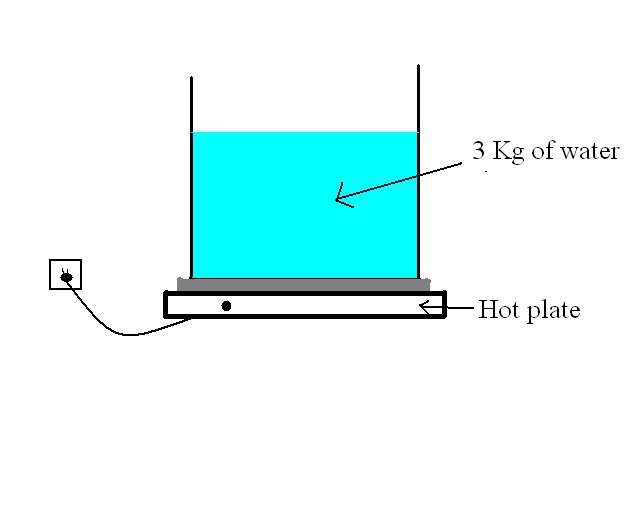# Heat power needed to partially evaporate water from a water container

In summary, the task at hand is to select a hot plate with a specific power rating that can evaporate 1 kg of water in an hour from a container containing 3 kg of water. The given equations and assumptions are used to calculate the necessary heat energy and the temperature of the water in the container. There is uncertainty about whether additional equations are needed to account for the presence of 3 kg of water in the container and the potential variation in temperature throughout the container.

## Homework Statement

So in the context of a practical project, I am tasked with selecting an appropriate hot plate with a specific power rating able to evaporate 1 kg of water in an hour, from a container that initially contains 3 kg of water.

## Homework Equations

- P = Q/Δt

- Q = mcwaterΔT with c= 4.18 J/(g.K)

- Q = mL with L=2260 J/g

- Electricity-to-heat energy conversion efficiency: assumed to be 100% to simplify the problem. So the power from the hot plate goes directly to the water in question.

- Water in the container is initially at 20 degrees Celsius.

- Reminder: the container doesn't contain only the 1 kg of water that evaporates, it also has a total of 3 kg of water.

- NO INFO whatsoever about the container's dimensions and constitution or the hot stove itself. The container is exposed to ambient air.

Here is an illustrative sketch for the problem:## The Attempt at a Solution

I first went like this:

P = Electrical energy/Δt = heat energy/Δt = heat energy/(3600 seconds)

Now my only problem is how to determine that heat energy. It would have been much easier if the container contained only the water to be evaporated, that is the 1 kg. In that case, it would have been:

Heat energy = mcwaterΔT + mL = 1000*4.18*(100-20) + 1000*2260
= some given number

Now I have no clue about what to do in the actual case, which is trying to evaporate 1kg of water from a total of 3 kg of water. Do I need extra equations in order to calculate that, or did I just misunderstand the concept ? It's been a while since my last Physics class ...